1. Worksheets>
2. Math>
3. Number Sense>
4. Estimation>
5. Decimal / Fraction

# Estimate the Decimals and Fractions Worksheets

Estimating decimals and fractions worksheets have skills to round the fractions to the nearest whole numbers and decimals to the nearest whole number or tenths and thereby estimating the sum, difference, product and quotients.

## Estimating Decimals

Rounding decimals

An exclusive page that contains enormous worksheets on rounding decimals to the nearest whole, tenths, hundredths and thousandths.

Rounding Decimals Worksheets

Estimate the sum / difference

Estimate the sum and difference by rounding each decimal number to the nearest whole number in the first three worksheets and to the nearest tenth in the remaining worksheets.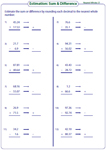nearest whole (horizontal):

nearest tenth (vertical):

MCQ: Estimating product

Choose the correct option which would provide the closest estimate for the given decimal multiplication problems.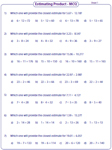Estimating Product

Estimating decimals worksheets have pages to round the whole numbers and the decimal numbers to its greatest place value and multiply to estimate the product.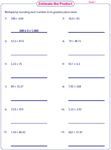MCQ: Estimating quotient

Estimating quotient worksheets have MCQs on dividing decimal numbers. Round each decimal number to find the appropriate quotient.Estimating quotient

Estimate the quotient by rounding the numerator and the denominator to any place value in such a way it leaves no remainder. Either the numerator or the denominator is a decimal number.## Estimating Fractions

Rounding fractions

An exclusive page that contains plentiful worksheets on rounding proper fraction, improper fraction and mixed number to the nearest whole number.

Rounding Fractions Worksheets

Estimating sum / difference

Each estimating fraction worksheet have practice problems on rounding mixed number to the nearest whole number and to thereby find the sum or difference.Estimate and compare

Round the mixed numbers to the nearest whole and estimate the product. Compare the product using greater than or less than symbol.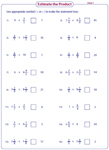Estimating Product

Estimate the product by rounding each mixed number to the nearest whole number.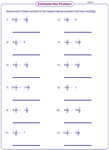Estimating Quotient

In these estimating quotient worksheets, round each mixed number to the nearest whole number and then divide to estimate the quotient. The dividend is always greater than the divisor.The entire collection of worksheets on estimating decimals and fractions can be downloaded in a jiffy!

Related Worksheets